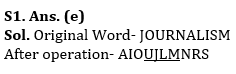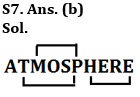Latest Banking jobs   »

# Reasoning Quizzes Quiz For IBPS RRB PO/Clerk Prelims 2023-02nd June

Q1. If all the letters in the word ‘JOURNALISM’ are arranged in alphabetical order from left to right in such a way that vowels are arranged first followed by consonants, then how many letters are in between O and N (in the word) after the arrangement?
(a) Two
(b) One
(c) None
(d) Three
(e) Four

Q2. If in the number ‘18397652’, 2 is subtracted from each of the digit which is greater than 6 and 1 is added to each of the digit which is less than 5 and three is added to each of the digit which is equal to 5 and 6, then how many digits are repeating in the number thus formed?
(a) Two
(b) One
(c) None
(d) Three
(e) Four

Q3. Mohit is 15th from the left end of a row and Ram is 22th from the right end of row. If they interchanged their positions then Ram ranks become 16th from right end. Find total number of persons in the row?
(a) 30
(b) 29
(c) 31
(d) 25
(e) Can’t be determined

Q4. Showing a woman on the stage, Ravi said, “She is the aunt of the son of only brother of my wife. How is the woman on stage related to Ravi?
(a) Wife
(b) Sister
(c) Mother-in-law
(d) Sister-in-law
(e) Mother

Q5. Which of the following will be definitely not true if the given expression
‘A > D ≥ R > J ≥ W > L = T ≤ C > B ≤ N’ is definitely true?
(a) R > T
(b) C ≥ T
(c) W < A
(d) L < D
(e) J ≥ T

Q6. Four of the following are alike in a certain way, so form a group, which among the following does not belong to that group?
(a) CEHL
(b) PRUY
(c) FHKO
(d) QSUX
(e) JLOS

Q7. How many pairs of letters are in the word ‘ATMOSPHERE’, each of which have as many letters between them as in the English alphabet?
(a) One
(b) Three
(c) Two
(d) Four
(e) None

Q8. If in the word ‘GEOGRAPHY’, all vowels are replaced by its next letter and all consonant is replaced by its previous letter, then all letters are arranged in alphabetical order from left to right. Then which of the following letter will be fourth from the right end?
(a) P
(b) B
(c) G
(d) O
(e) F

Q9. If the number ‘53982764’, One is subtracted from odd digits and one is added to even digits of the number, then digits are arranged in ascending order from left to right. Then what will be the sum of the digit which is third from the left end and the digit which is fourth from the right end?
(a) 8
(b) 12
(c) 10
(d) 5
(e) None of these

Q10. How many meaningful words can be formed from the 2nd, 3rd, 5th and 7th letter of a word ‘OPTIMISM’ by using each letter once in the word?
(a) Three
(b) One
(c) None
(d) Two
(e) More than three

SolutionsS2. Ans. (c)
Sol. Original number- 18397652
Obtained number- 26475983

S3. Ans (a)
Sol. Total number of persons in the row (by using Ram’s rank from both side) = (15+16-1) =30

S4. Ans. (a)
S5. Ans. (e)
S6. Ans. (d)S8. Ans. (d)
Sol. Final word after both operation = BFFFGOOQX

S9. Ans. (c)
Sol. Final number after operation = 23456789
Required sum = 4 + 6 = 10

S10. Ans. (c)
Sol. 2nd, 3rd, 5th and 7th letter = P, T, M, S
No meaningful words can be formed.## FAQs

### What is the selection process of IBPS RRP PO?

There are 4 sections in the IRDAI assistant manager online preliminary exam. They are Reasoning, English Language, General awareness, Quantitative aptitude.

#### Congratulations!Union Budget 2023-24: Free PDF